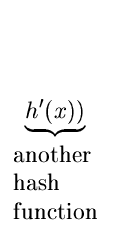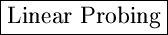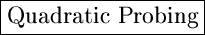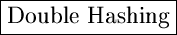Next:3.4.2 An Example:Up:3.4 Closed HashingPrevious:3.4 Closed Hashing

## 3.4.1 Rehashing Methods

Denote h(x, 0) by simply h(x).
1.
Linear probing
h(x, i) = (h(x) + i) mod m
2.
h(x, i) = (h(x) + C1i + C2i2) mod m

where C1 and C2 are constants.
3.
Double Hashing
h(x, i) = (h(x) + imod m

A Comparison of Rehashing Methodsm distinct probe Primary clustering sequencesm distinct probe No primary clustering; sequences but secondary clusteringm2 distinct probe No primary clustering sequences No secondary clustering

eEL,CSA_Dept,IISc,Bangalore